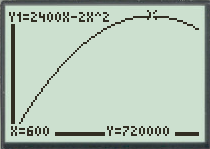# The dimensions of the field of largest area that a farmer can fence.### Precalculus: Mathematics for Calcu...

6th Edition
Stewart + 5 others
Publisher: Cengage Learning
ISBN: 9780840068071### Precalculus: Mathematics for Calcu...

6th Edition
Stewart + 5 others
Publisher: Cengage Learning
ISBN: 9780840068071

#### Solutions

Chapter 3.1, Problem 71E
To determine

## To find: The dimensions of the field of largest area that a farmer can fence.

Expert Solution

The dimensions of the field of largest area that the farmer can fence is 600ft by 1200ft .

### Explanation of Solution

Given information:

A farmer has 2400ft of fencing and wants to fence off a rectangular field that borders a straight river. The farmer doesn’t fence along the river.

Calculation:

As per given, assume that the length of the rectangular field is equal to xft .

As the length is fenced two times i.e. length of the fence for these two sides is equal to 2xft and as the total fence is 2400ft . So, the breadth of the rectangular field is equal to 24002xft .

Use the formula for the area of the rectangular region.

A=L×BA=x×(24002x)A=2400x2x2

So, the area function of the rectangular region is given by g(x)=2400xx2 . To maximize the area of the rectangular region graph the area function g(x)=2400xx2 .

To graph a function y=2400x2x2 , follow the steps using graphing calculator.

First press “ON” button on graphical calculator, press Y= key and enter right hand side of the equation y=2400x2x2 after the symbol Y1 . Enter the keystrokes 2400X2X^2 .

The display will show the equation,

Y1=2400X2X^2

Now, press the GRAPH key and TRACE key to produce the graph of given function in standard window as shown in Figure (1).Figure (1)

As observed from the graph, the area function has maximum value at x=600 .

So, the length of the rectangular region is equal to 600ft and the breadth of the rectangular region is equal to 24002(600)=1200ft .

Therefore, the dimensions of the field of largest area that the farmer can fence is 600ft by 1200ft .

### Have a homework question?

Subscribe to bartleby learn! Ask subject matter experts 30 homework questions each month. Plus, you’ll have access to millions of step-by-step textbook answers!